Dr. Herrick's CH223 Pressure Quiz 7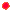Electrochemistry Copyright  D. Herrick20 Questions from past exams. Practice for speed.  Aim for 2 minutes per problem.
 General Information:  *Faraday constant:     F = 96,500 Coulomb / mol *Gas constant:           R = 8.314 J / mol-deg *Volt conversion:        1 V = 1 J / Coulomb *The symbol E denotes EMF. *All reactions are at 25C = 298K.
 1 Given the half-reactions Sn2+(aq) + 2e–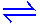Sn(s) , Eo = -0.136 V AgCl(s) +  e–Ag(s) + Cl–(aq) , Eo = 0.222 V calculate standard-state EMF (Eo) for an electrochemical cell based on the reaction Sn(s) + 2AgCl(s)2Ag(s) + 2Cl–(aq) + Sn2+(aq)
 A) 0.580V B) 0.358 C) 0.086 D) -0.358 E) -0.580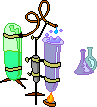2 In a working electrochemical cell, reduction occurs at the
 A) anode B) membrane barrier C) catalyst D) acidic electrode E) cathode
 3 Which statement is FALSE?
 A) The species that gains electrons in an electrochemical reaction is reduced. B) By convention, the half-reaction  2H+(aq) + 2e–H2(g) has a standard EMF of Eo = 0. C) The species that loses electrons in an electrochemical reaction is oxidized. D) The standard state of O2(g) has P = 1 atm. E) The standard state of Cu2+(aq) has [Cu2+] = 1 M. F) The reaction quotient Q satisfies Q = 1 when reactants and products have their standard-state concentrations or pressures. G) The reaction quotient satisfies Q = K when all reactants and products have their equilibrium concentrations or pressures. H) K in the preceding answer (G) is the equilibrium constant expression. I) The free-energy change for a chemical reaction with reactants and products at arbitrary concentrations or pressures is given by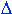G =Go + RT ln(Q)  =  RT ln(Q/K) . This means the spontaneous direction of reaction favors formation of more products when Q < K, and more reactants when Q > K.  Some students prefer to write these as K > Q and K < Q so that the symbols > and < point in the direction of spontaneous reaction. J) The Nernst equation for the EMF (E, in Volts) generated by an electrochemical cell at 25C is derived from the free-energy change to be E = Eo - (0.0592/ne) log(Q) where "log" refers to base 10 (ex:  log(100) = 2). K) ne in the preceding answer (J) is the number of mols of electrons transferred in the balanced oxidation-reduction reaction. L) The EMF of a spontaneous electrochemical reaction is positive. M) The maximum possible work from a voltaic cell is obtained when the current is drawn very slowly. N) Since the EMF of an electrochemical reaction satisfies E = 0 at equilibrium, the equilibrium constant for the reaction at 25C can be determined from the Nernst equation in the form log(K)  =  ne Eo / 0.0592 when Eo is given in Volts. O) none of the above4 Find the value of ne for a voltaic cell based on oxidation-reduction half-reactions for the combustion reaction:  CH4 + 2 O2CO2 +  2 H2O
 A) ne= 1 F) ne= 6 B) ne= 2 G) ne= 7 C) ne= 3 H) ne= 8 D) ne= 4 I) ne= 9 E) ne= 5 J) ne= 10
 5 In a working electrochemical cell oxidation occurs at the
 A) anode B) surface of the liquid C) catalyst D) acidic electrode E) cathode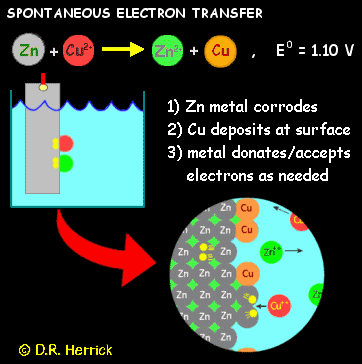6 The role of Cu2+ in the electron transfer reaction  Zn + Cu2+Zn2+ + Cu    is
 A) oxidizing agent B) spectator ion C) reducing agent D) catalyst
 7 The FALSE statement about a working electrochemical cell is:
 A) The anode is the electrode at which oxidation of chemical species occurs. B) Cations and anions--not electrons--conduct the electrical current in solution. C) The EMF of a voltaic cell is independent of the temperature. D) Aqueous ions with positive charges migrate toward the cathode.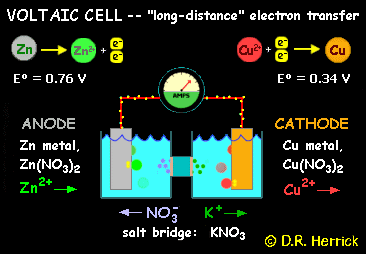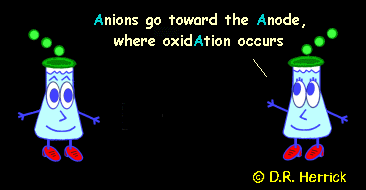Data for Questions 8-11: Cr3+(aq) + 3e–Cr(s) ,    Eo = -0.74 V Fe2+(aq) + 2e–Fe(s) ,    Eo = -0.41 V Mg2+(aq) + 2e–Mg(s) ,  Eo = -2.38 V Sn2+(aq) + 2e–Sn(s) ,   Eo = -0.14 V
 8 The strongest oxidizing agent is:
 A) Fe2+ E) Sn2+ B) Cr3+ F) Mg2+ C) Sn G) Fe D) Cr H) Mg
 9 The strongest reducing agent is:
 A) Fe2+ E) Sn2+ B) Cr3+ F) Mg2+ C) Sn G) Fe D) Cr H) Mg
 10 Find the standard EMF (Eo) of the reaction 3Sn(s) + 2Cr3+(aq)2Cr(s) + 3Sn2+(aq)
 A) -1.76 V F) 0.44 B) -1.20 G) 0.6 C) -0.88 H) 0.88 D) -0.60 I) 1.2 E) -0.44 J) 1.76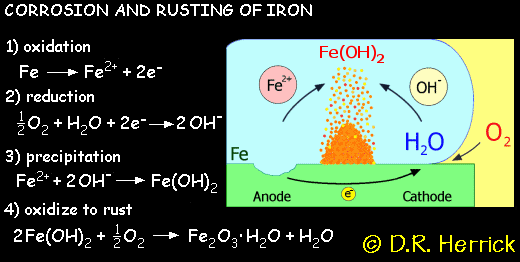11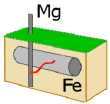Magnesium metal attached to a piece of iron  acts as a "sacrificial anode" that inhibits rusting because Mg loses electrons more readily than Fe.  Calculate Eo for the reaction Mg(s) + Fe2+(aq)Mg2+(aq) + Fe(s)
 A) 2.79 V B) 1.97 C) 0 D) -1.97 E) -2.79
 12 When Cu is obtained by electrolysis from CuSO4 , the minimum number of coulombs required to produce 1.25 mol of copper is
 A) 38,600 B) 77,200 C) 60,312 D) 120,625 E) 241,250
 13 When Al is obtained by electrolysis from Al2O3 , the number of coulombs required to produce 1.00 mol of aluminum is
 A) 32,167 B) 64,333 C) 193,000 D) 289,500 E) 579,00014 Calculate Eo for the reaction 2Al(s) + 3Fe2+(aq)2Al3+(aq) + 3Fe(s) given Fe2+(aq) + 2e–Fe(s) ,  Eo = -0.41 V Al3+(aq) + 3e–Al(s),    Eo = -1.66 V
 A) 2.09V B) 1.25 C) zero D) -1.25 E) -2.09
 15 What is ne in the Nernst equation for the reaction in Question 14?
 A) 3 B) 2 C) 5 D) 6 E) 4
 16 Use the Nernst equation to determine the emf for a voltaic cell based on the reaction Cl2(g, 1atm) + 2I– (aq, 2.00M)2Cl– (aq, 0.02M) + I2(s), Eo = 0.83 V
 A) 1.07V B) 0.59 C) 0.71 D) 1.66 E) 0.95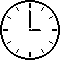17 Use the Nernst equation to determine the emf for a voltaic cell based on the reaction Cr(s) + 3 Ag+(0.00010 M)Cr3+(0.010 M) + 3 Ag(s), Eo = 1.540 V
 A) 1.34V B) 1.29 C) 1.74 D) 1.54 E) 1.88
 18 Find the equilibrium constant for the reaction O2(g) + 4H+(aq) + 4Fe2+(aq)4Fe3+(aq) + 2 H2O(l ) , Eo = 0.460V
 A) 5.9 × 107 B) 3.6 × 1016 C) 8.1 × 10-32 D) 1.2 × 1031 E) 8.3 × 1028
 19 Calculate the EMF generated by a voltaic cell operating on the reaction Zn(s) + 2H+(aq, pH=3)Zn2+(aq, 1M) + H2(g, 1 atm), Eo = 0.76 V
 A) 1.12V B) 0.46 C) 0.67 D) 0.58 E) 0.94
 20 Find the equilibrium constant of the reaction Fe(s) + Cd2+(aq)Fe2+(aq) + Cd(s), Eo = 0.0400V
 A) 22.5 B) 4.7 C) 0.045 D) 0.21 E) 1.00